Copyright (C) 2012-present, The Computational Data Science Lab
https://github.com/cdslaborg/paramonte
References

# Simple power-law (or linear!) regression via the ParaDRAM sampler

NOTE
If you are viewing an HTML version of this MATLAB live script on the web, you can download the corresponding MATLAB live script *.mlx file to this HTML page at,
This code, along with other MATLAB live scripts, is located at,
https://github.com/cdslaborg/paramontex/tree/main/MATLAB/mlx
Once you download the file, open it in MATLAB to view and interact with its contents, which is the same as what you see on this page.
NOTE
This ParaDRAM sampler example is also available in Python language as a Jupyter Notebook, on this page,
This Jupyter Notebook, along with other Python Jupyter Notebooks, is located at,
https://github.com/cdslaborg/paramontex/tree/main/Python/Jupyter
IMPORTANT - macOS users
If you are a macOS (Darwin) user and you have downloaded the prebuilt ParaMonte libraries from the GitHub release page to use on your macOS system, read this troubleshooting page before continuing.

## Setting up the path to the ParaMonte::MATLAB library

================================================================
First, we will clean up the MATLAB environment and make sure the path to the ParaMonte library is in MATLAB's path list.
clc;
clear all;
close all;
format compact; format long;
%%%%%%%%%%%% IMPORTANT %%%%%%%%%%%%%
% Set the path to the ParaMonte library:
% Change the following path to the ParaMonte library root directory,
% otherwise, make sure the path to the ParaMonte library is already added
% to MATLAB's path list.
pmlibRootDir = './';
%%%%%%%%%%%%%%%%%%%%%%%%%%%%%%%%%%%%
% change MATLAB's working directory to the folder containing this script
% if MATLAB Live Scripts did not create a temporary folder, we would not
% have all of these problems!
try
setwdFilePath = websave("setwd.m","https://github.com/cdslaborg/paramontex/raw/main/MATLAB/mlx/setwd.m");
run(setwdFilePath); % This is a MATLAB script that you can download from the same GitHub location given in the above.
catch % alas, we will have to run the simulations in MATLAB Live Script's temporary folder
filePath = mfilename('fullpath');
[currentDir,fileName,fileExt] = fileparts(filePath); cd(currentDir);
cd(fileparts(mfilename('fullpath'))); % Change working directory to source code directory.
end

## Simple power-law (or linear!) regression

===============================================
Suppose we have collected a dataset comprised of following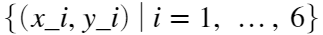pair observations,

####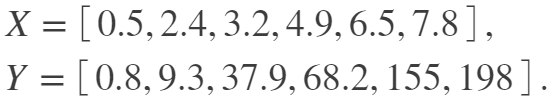and we would like to model the dependence of Y on X. First thing to do in such problems is to explore the data and visualize it in search of any potential patterns, as we do below.
X = [0.5, 2.4, 3.2, 4.9, 6.5, 7.8];
Y = [0.8, 9.3, 37.9, 68.2, 155, 198];
figure; hold on; box on;
plot(X,Y,".", "markerSize", 30, "color","red");
xlabel("X");
ylabel("Y");
set(gca,"xscale","linear","yscale","linear");
hold off;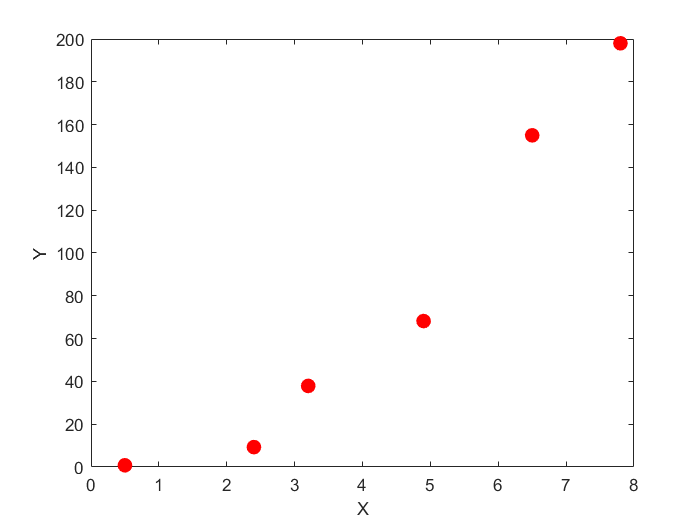Obviously, the relationship between X and Y in this dataset is non-linear. A fairly experienced Data Scientist could immediately guess the underlying relatioship in this dataset: power-law, which can be readily confirmed by checking the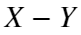relationship on a log-log plot. This works because if,

####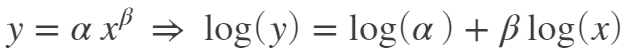figure; hold on; box on;
plot(X,Y,".", "markerSize", 30, "color","red");
xlabel("X");
ylabel("Y");
set(gca,"xscale","log","yscale","log");
hold off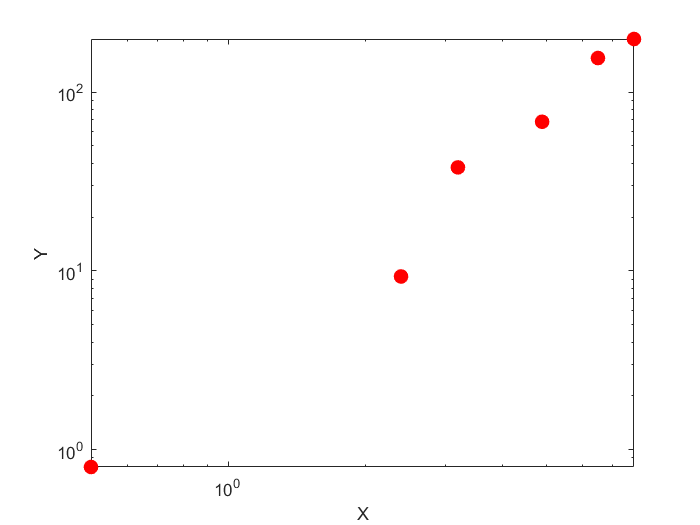However, a simple line does not perfectly fit the (log-transformed) data either. Therefore, we must also take into account the inherent variability in our data around the linear fit. Although not always correct, the simplest most popular assumption about the noise in data is that the Y is distributed according to a Normal distribution around the best-fit line to our log-transformed data,

####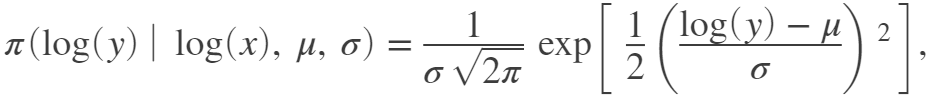The unknown parameters of this Normal distribution, the mean ( μ ) and the standard deviation ( σ ) would have to be then determined by fitting. Fortunately, the mean of the normal distribution can be simply taken to be the prediction of our best linear fit. Therefore, the mean is a function of the x value and the slope and intercept of our linear fit to log-transformed data. However, the standard deviation would be to fit and constrained separately.
Now that we have constructed our model and the noise, we can proceed to use the principle of Maximum Likelihood to constrain the unknown parameters of the model using the Markov Chain Monte Carlo approach that is available from the ParaMonte library via the ParaDRAM() sampler class.
To summarize, the unknown parameters are the slope and intercept of the linear fit and the standard deviation sigma of the Normal distribution.
The probability of observing a single data point is given by the above Normal Probability Density Function (PDF). Therefore, the probability of observing all of our dataset, assuming they are independent and identically distributed (i.i.d.), is simply the multiplication of all of the individual probabilities,

####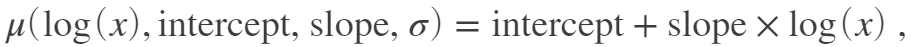The likelihood is often difficult as the likelihood value is often very tiny causing underflow in computers. Therefore, we often prefer to work with the log-transformation of the likelihood which does not suffer from the same problem,

####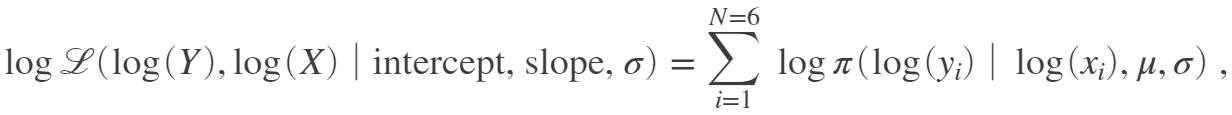We now go on to implement this log-likelihood as a MATLAB function. However, before doing so, we shall take care of an extremely important issue: The possible range of values of the parameters.
If you pay attention to the parameters, you will notice that the intercept and slope can in general be any real value, from negative infinity to positive infinity. However, the third parameter, the standard deviation of the Normal distribution, is a strictly positive-valued number. This range-asymmetry can cause instabilities in our Markov Chain sampler.
How can we solve this and symmetrize the range of sigma? The answer is simple: We will sample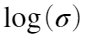instead of σ itself. So, our third sampling parameter will be logSigma instead of sigma, which has a symmetric possible range from negative infinity to positive infinity. Then, inside our likelihood function, we will convert logSigma back to sigma.
The getLogLike() function implementation is provided at the end of this live script. Now let's test this likelihood function for a random input test value for the parameters to ensure it works fine. We will pick our best guess (simply by visual inspection of the data log-log plot).
getLogLike([0.8,2,0.1])
ans = -6.501444916760644
NOTE
Since the mathematical objective functions (e.g., probability density functions) can take extremely small or large values, we often work with their natural logarithms instead. This is the reason behind the naming convention used in the ParaMonte library for the user's objective functions: getLogFunc, implying that the user must provide a function that returns the natural logarithm of the target objective function.
See MATLAB Live Script for an in-depth discussion of why we need to work with the logarithm of mathematical objective functions in optimization and sampling problems.
IMPORTANT
Note that in MATLAB all vectors and arrays are, by default, column-major, and so is the input value, point, to getLogFunc(). This means that the size of point is (ndim,1). Thus, all other vectors that potentially interact with point, must be also of the same size as point: (ndim,1).

## Running a ParaDRAM simulation on a single processor

================================================================
We can now proceed to the set up a ParaDRAM Markov Chain Monte Carlo sampler to constrain the three unknown parameters of the model.
Note: To run the ParaMonte samplers in parallel, visit the ParaMonte library's documentation website.
We will first create an instance of the paramonte class,
pm = paramonte();

### Checking the ParaMonte library's version

==========================================
Before runnnig any simulations, it is good to check which version of the ParaMonte library you have and are working with,
pm.version.interface.get() % get the ParaMonte::MATLAB (interface) version
ans = "ParaMonte MATLAB Interface Version 2.5.0"
pm.version.interface.dump()
ans = "2.5.0"
pm.version.kernel.get() % the ParaMonte::Kernel version (the version of the ParaDRAM sampler)
ans = "ParaMonte MATLAB Kernel Version 1.5.1"
pm.version.kernel.dump()
ans = "1.5.1"
We can also verify the existence of the essential components of the current installation of the ParaMonte library on the system,
pm.verify();
::::::::::::::::::::::::::::::::::::::::::::::::::::::::::::::::::::::::::::::::::::::::::::::: :::: :::: _/_/_/_/ _/_/ _/_/ _/ _/ _/_/_/_/_/ _/ _/ _/ _/_/_/_/ _/ /_/_/ _/_/_/_/ _/ _/ _/ _/_/ _/_/_/ _/_/_/ _/_/_/ _/_/_/ _/ _/ _/_/ _/ _/ _/ _/ _/ _/ _/ _/ _/ _/_/_/_/ _/ _/ _/ _/ _/ _/ _/ _/ _/ _/ _/ _/ _/ _/ _/_/_/ _/_/_/_/ _/ _/_/_/_/ _/_/_/ _/_/_/ _/_/ _/ _/ _/_/ _/_/_/ ParaMonte plain powerful parallel Monte Carlo library Version 2.5.0 :::: :::: ::::::::::::::::::::::::::::::::::::::::::::::::::::::::::::::::::::::::::::::::::::::::::::::: ParaMonte - NOTE: Intel MPI library for 64-bit architecture detected at: ParaMonte - NOTE: ParaMonte - NOTE: "C:\Program Files (x86)\IntelSWTools\compilers_and_libraries_2020.1.216\windows\mpi\intel64\bin" ParaMonte - NOTE: ParaMonte - NOTE: To perform ParaMonte simulations in parallel on a single node, run the ParaMonte - NOTE: following two commands, in the form and order specified, on a MATLAB-aware ParaMonte - NOTE: mpiexec-aware command-line interface, such as the Intel Parallel Studio's command-prompt: ParaMonte - NOTE: ParaMonte - NOTE: "C:\Program Files (x86)\IntelSWTools\compilers_and_libraries_2020.1.216\windows\mpi\intel64\bin\mpivars.bat" ParaMonte - NOTE: ParaMonte - NOTE: mpiexec -localonly -n 3 matlab -batch "main_mpi" ParaMonte - NOTE: ParaMonte - NOTE: where, ParaMonte - NOTE: ParaMonte - NOTE: 0. the first command defines the essential environment variables and, ParaMonte - NOTE: the second command runs in the simulation in parallel, in which, ParaMonte - NOTE: 1. you should replace the default number 3 with the number of ParaMonte - NOTE: processors you wish to assign to your simulation task, ParaMonte - NOTE: 2. main_mpi.m is the MATLAB file which serves as the entry point to ParaMonte - NOTE: your simulation, where you call the ParaMonte sampler routines. ParaMonte - NOTE: ATTN: Notice the MATLAB filename must appear without the file extension. ParaMonte - NOTE: ATTN: Notice the MATLAB filename must be enclosed with quotation marks. ParaMonte - NOTE: 3. -localonly indicates a parallel simulation on only a single node (this ParaMonte - NOTE: flag will obviate the need for MPI library credentials registration). ParaMonte - NOTE: For more information, visit: ParaMonte - NOTE: ParaMonte - NOTE: https://software.intel.com/en-us/get-started-with-mpi-for-windows ParaMonte - NOTE: ParaMonte - NOTE: Note that the above two commands must be executed on a command-line that recognizes ParaMonte - NOTE: both MATLAB and mpiexec applications, such as the Intel Parallel Studio's command-prompt. ParaMonte - NOTE: For more information, in particular, on how to register to run Hydra services ParaMonte - NOTE: for multi-node simulations on Windows servers, visit: ParaMonte - NOTE: ParaMonte - NOTE: https://www.cdslab.org/paramonte ParaMonte - NOTE: To check for the ParaMonte or the MPI library installations status or, ParaMonte - NOTE: to display the above messages in the future, use the following ParaMonte - NOTE: commands on the MATLAB command-line: ParaMonte - NOTE: ParaMonte - NOTE: pm = paramonte(); ParaMonte - NOTE: pm.verify();

### Setting up the ParaDRAM simulation specifications

====================================================
Now, we create an instance of the ParaDRAM sampler class,
The simplest scenario is to run the simulation with the default specifications that are appropriately determined by the ParaDRAM sampler. However, for the purpose of clarity reproducibility, we will specify a few simulation specs,
For a complete list of all ParaDRAM simulation specifications, visit the ParaDRAM simulation specifications webpage.

#### The simulation output file names and storage path

We will store all output files in a directory with the same name as this MATLAB Live Script's name.
By default, all ParaDRAM simulation output files are named properly (see this page for a review of ParaDRAM simulation output files). In general, it is a good habit to specify an output file prefix for any ParaDRAM simulation. This way, the restart of an incomplete simulation, if it happens for some reason, would be seamless and doable by just rerunning the simulation, without any change of any sort to any parts of the codes or the simulation.
We will prefix all simulation output files the same as the filename of this script,
pmpd.spec.outputFileName = "./out/regression_powerlaw"; % specify the output file prefixes.
pmpd.spec.helpme("outputFileName");
The above outputFileName will result in the generation of a folder named ./out/, which will keep the simulation output files, all of which are prefixed with mvn_serial.
NOTE
If the specified prefix ends with a forward slash / on Linux/macOS or with a backslash \ on Windows, then the prefix provided will serve as the directory in which the output files will be stored.

#### Ensuring the reproducibility of the results

We will also initialize the random seed to a fixed number to ensure the reproducibility of the simulation results in the future,
pmpd.spec.randomSeed = 12345; % set the random seed for the sake of reproducibity.
pmpd.spec.helpme("randomSeed");
For the sake of illustration, let's also ask for a modest refinement (decorrelation) of the final sample,
pmpd.spec.overwriteRequested = true; % overwrite the existing output files just in case they already exist.
pmpd.spec.variableNameList = ["intercept", "slope", "logSigma"]; % set the output names of the parameters.
pmpd.spec.chainSize = 20000; % set the number of uniquely sampled points from the likelihood function.
Since we have three unknown parameters to estimate (the intercept and slope of the linear fit as well as the dispersion of data around the fit as measured by the standard deviation of the Normal distribution ( σ ), we will define NDIM = 3.
NDIM = 3; % The number of unknown parameters to constrain.

#### The default domain of the objective function

The default starting point of the sampler for exploring the objective function is the center of the domain of the objective function. The default domain of the objective function chosen by the sampler is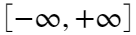along each dimension of the objective function. This is a fine default assumption for this particular simulation and in most other scenarios. If needed, you can change them via the following attributes,
pmpd.spec.domainLowerLimitVec = -1.e300 * ones(NDIM,1); % set the lower limits of the domain of the objective function to negative infinity (This is the default value)
pmpd.spec.domainUpperLimitVec = +1.e300 * ones(NDIM,1); % set the upper limits of the domain of the objective function to negative infinity (This is the default value)
The ParaDRAM sampler guarantees to not sample any points outside the hypercube defined by the above limits.

===============================
Note that none of the above specification settings were necessary, but is in general a good habit to define them prior to the simulation.
We now call the ParaDRAM sampler routine to run the sampling simulation. For the same of this simulation, we will also make the output files overwritable (before doing, The ParaDRAM sampler method takes only two arguments. We can call it like the following,
pmpd.runSampler ( NDIM ... This is the number of dimensions of the objective function
, @getLogLike ... This is the objective function
);
• On Windows operating systems (OS), the sampler will print the realtime simulation progress information on your MATLAB prompt window.
• On macOS / Linux OS, the sampler will print the realtime simulation progress information on the Bash terminal from which you invoked the MATLAB executable to open the MATLAB application.
Done. We can read the generated sample to analyze and visualize the results. However, before doing so, we will make sure there is no lack of convergence in the MCMC chain by inspecting the **adaptation measure** of the chain that is available in the output chain file.
ParaDRAM - WARNING: The ParaDRAM input simulation specification pmpd.spec.outputDelimiter is not set. ParaDRAM - WARNING: This information is essential for successful reading of the requested chain file(s). ParaDRAM - WARNING: Proceeding with the default assumption of comma-delimited chain file contents... ParaDRAM - NOTE: 1 files detected matching the pattern: ParaDRAM - NOTE: "D:\Dropbox\Projects\20180101_ParaMonte\git\paramontex\MATLAB\mlx\regression_powerlaw_data_paradram\out\regression_powerlaw*" ParaDRAM - NOTE: processing file: "D:\Dropbox\Projects\20180101_ParaMonte\git\paramontex\MATLAB\mlx\regression_powerlaw_data_paradram\out\regression_powerlaw_process_1_chain.txt" ParaDRAM - NOTE: reading the file contents... ParaDRAM - NOTE: done in 0.707380 seconds. ParaDRAM - NOTE: ndim = 3, count = 20000 ParaDRAM - NOTE: computing the sample correlation matrix... ParaDRAM - NOTE: creating the heatmap plot object from scratch... ParaDRAM - NOTE: done in 0.316870 seconds. ParaDRAM - NOTE: computing the sample covariance matrix... ParaDRAM - NOTE: creating the heatmap plot object from scratch... ParaDRAM - NOTE: done in 0.126480 seconds. ParaDRAM - NOTE: computing the sample autocorrelation... ParaDRAM - NOTE: creating the line plot object from scratch... ParaDRAM - NOTE: creating the scatter plot object from scratch... ParaDRAM - NOTE: creating the lineScatter plot object from scratch... ParaDRAM - NOTE: done in 0.538260 seconds. ParaDRAM - NOTE: adding the graphics tools... ParaDRAM - NOTE: creating the line plot object from scratch... ParaDRAM - NOTE: creating the scatter plot object from scratch... ParaDRAM - NOTE: creating the lineScatter plot object from scratch... ParaDRAM - NOTE: creating the line3 plot object from scratch... ParaDRAM - NOTE: creating the scatter3 plot object from scratch... ParaDRAM - NOTE: creating the lineScatter3 plot object from scratch... ParaDRAM - NOTE: creating the histogram plot object from scratch... ParaDRAM - NOTE: creating the histogram2 plot object from scratch... ParaDRAM - NOTE: creating the histfit plot object from scratch... ParaDRAM - NOTE: creating the contour plot object from scratch... ParaDRAM - NOTE: creating the contourf plot object from scratch... ParaDRAM - NOTE: creating the contour3 plot object from scratch... ParaDRAM - NOTE: creating the grid plot object from scratch... ParaDRAM - NOTE: The processed chain files are now stored in the output variable as a cell array. For example, to ParaDRAM - NOTE: access the contents of the first (or the only) chain file stored in an output variable named ParaDRAM - NOTE: OUTPUT_CELL_ARRAY, try: ParaDRAM - NOTE: ParaDRAM - NOTE: OUTPUT_CELL_ARRAY{1}.df ParaDRAM - NOTE: ParaDRAM - NOTE: To access the plotting tools, try: ParaDRAM - NOTE: ParaDRAM - NOTE: OUTPUT_CELL_ARRAY{1}.plot.<PRESS TAB TO SEE THE LIST OF PLOTS> ParaDRAM - NOTE: ParaDRAM - NOTE: For example, ParaDRAM - NOTE: ParaDRAM - NOTE: OUTPUT_CELL_ARRAY{1}.plot.line.make() % to make 2D line plots. ParaDRAM - NOTE: OUTPUT_CELL_ARRAY{1}.plot.scatter.make() % to make 2D scatter plots. ParaDRAM - NOTE: OUTPUT_CELL_ARRAY{1}.plot.lineScatter.make() % to make 2D line-scatter plots. ParaDRAM - NOTE: OUTPUT_CELL_ARRAY{1}.plot.line3.make() % to make 3D line plots. ParaDRAM - NOTE: OUTPUT_CELL_ARRAY{1}.plot.scatter3.make() % to make 3D scatter plots. ParaDRAM - NOTE: OUTPUT_CELL_ARRAY{1}.plot.lineScatter3.make() % to make 3D line-scatter plots. ParaDRAM - NOTE: OUTPUT_CELL_ARRAY{1}.plot.contour3.make() % to make 3D kernel-density contour plots. ParaDRAM - NOTE: OUTPUT_CELL_ARRAY{1}.plot.contourf.make() % to make 2D kernel-density filled-contour plots. ParaDRAM - NOTE: OUTPUT_CELL_ARRAY{1}.plot.contour.make() % to make 2D kernel-density plots. ParaDRAM - NOTE: OUTPUT_CELL_ARRAY{1}.plot.histogram2.make() % to make 2D histograms. ParaDRAM - NOTE: OUTPUT_CELL_ARRAY{1}.plot.histogram.make() % to make 1D histograms. ParaDRAM - NOTE: OUTPUT_CELL_ARRAY{1}.plot.grid.make() % to make GridPlot ParaDRAM - NOTE: ParaDRAM - NOTE: To plot or inspect the variable autocorrelations or the correlation/covariance matrices, try: ParaDRAM - NOTE: ParaDRAM - NOTE: OUTPUT_CELL_ARRAY{1}.stats.<PRESS TAB TO SEE THE LIST OF COMPONENTS> ParaDRAM - NOTE: ParaDRAM - NOTE: For more information and examples on the usage, visit: ParaDRAM - NOTE: ParaDRAM - NOTE: https://www.cdslab.org/paramonte
chain = chainList{1}; % Choose the contents of the first read chain file (in this case, there is only one file).
chain.plot.scatter.ycolumns = "AdaptationMeasure"; ... Choose the AdaptationMeasure column of the output chain file to plot.
chain.plot.scatter.ccolumns = []; % Set the color of the points to empty from the default logLikelihood value.
chain.plot.scatter.make();
set(gca,"yscale","log");
xlabel("MCMC Sample Count");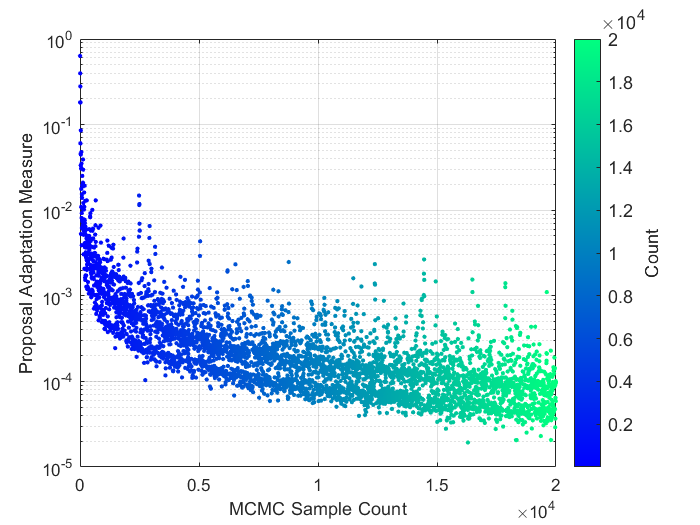The power-law decay of the adaptation measure tells us everything has gone well with our simulation. If there were to exist any signs of a lack of convergence, we would not see this power-law decaying behavior. This power-law decay implies that the proposal adaptation decayed and diminished consistently throughout the entire simulation, as we wished.
We can now visualize the resulting refined sample by reading and plotting it from the output sample file.
ParaDRAM - WARNING: The ParaDRAM input simulation specification pmpd.spec.outputDelimiter is not set. ParaDRAM - WARNING: This information is essential for successful reading of the requested sample file(s). ParaDRAM - WARNING: Proceeding with the default assumption of comma-delimited sample file contents... ParaDRAM - NOTE: 1 files detected matching the pattern: ParaDRAM - NOTE: "D:\Dropbox\Projects\20180101_ParaMonte\git\paramontex\MATLAB\mlx\regression_powerlaw_data_paradram\out\regression_powerlaw*" ParaDRAM - NOTE: processing file: "D:\Dropbox\Projects\20180101_ParaMonte\git\paramontex\MATLAB\mlx\regression_powerlaw_data_paradram\out\regression_powerlaw_process_1_sample.txt" ParaDRAM - NOTE: reading the file contents... ParaDRAM - NOTE: done in 0.041740 seconds. ParaDRAM - NOTE: ndim = 3, count = 3033 ParaDRAM - NOTE: computing the sample correlation matrix... ParaDRAM - NOTE: creating the heatmap plot object from scratch... ParaDRAM - NOTE: done in 0.076205 seconds. ParaDRAM - NOTE: computing the sample covariance matrix... ParaDRAM - NOTE: creating the heatmap plot object from scratch... ParaDRAM - NOTE: done in 0.024757 seconds. ParaDRAM - NOTE: computing the sample autocorrelation... ParaDRAM - NOTE: creating the line plot object from scratch... ParaDRAM - NOTE: creating the scatter plot object from scratch... ParaDRAM - NOTE: creating the lineScatter plot object from scratch... ParaDRAM - NOTE: done in 0.142410 seconds. ParaDRAM - NOTE: adding the graphics tools... ParaDRAM - NOTE: creating the line plot object from scratch... ParaDRAM - NOTE: creating the scatter plot object from scratch... ParaDRAM - NOTE: creating the lineScatter plot object from scratch... ParaDRAM - NOTE: creating the line3 plot object from scratch... ParaDRAM - NOTE: creating the scatter3 plot object from scratch... ParaDRAM - NOTE: creating the lineScatter3 plot object from scratch... ParaDRAM - NOTE: creating the histogram plot object from scratch... ParaDRAM - NOTE: creating the histogram2 plot object from scratch... ParaDRAM - NOTE: creating the histfit plot object from scratch... ParaDRAM - NOTE: creating the contour plot object from scratch... ParaDRAM - NOTE: creating the contourf plot object from scratch... ParaDRAM - NOTE: creating the contour3 plot object from scratch... ParaDRAM - NOTE: creating the grid plot object from scratch... ParaDRAM - NOTE: The processed sample files are now stored in the output variable as a cell array. For example, to ParaDRAM - NOTE: access the contents of the first (or the only) sample file stored in an output variable named ParaDRAM - NOTE: OUTPUT_CELL_ARRAY, try: ParaDRAM - NOTE: ParaDRAM - NOTE: OUTPUT_CELL_ARRAY{1}.df ParaDRAM - NOTE: ParaDRAM - NOTE: To access the plotting tools, try: ParaDRAM - NOTE: ParaDRAM - NOTE: OUTPUT_CELL_ARRAY{1}.plot.<PRESS TAB TO SEE THE LIST OF PLOTS> ParaDRAM - NOTE: ParaDRAM - NOTE: For example, ParaDRAM - NOTE: ParaDRAM - NOTE: OUTPUT_CELL_ARRAY{1}.plot.line.make() % to make 2D line plots. ParaDRAM - NOTE: OUTPUT_CELL_ARRAY{1}.plot.scatter.make() % to make 2D scatter plots. ParaDRAM - NOTE: OUTPUT_CELL_ARRAY{1}.plot.lineScatter.make() % to make 2D line-scatter plots. ParaDRAM - NOTE: OUTPUT_CELL_ARRAY{1}.plot.line3.make() % to make 3D line plots. ParaDRAM - NOTE: OUTPUT_CELL_ARRAY{1}.plot.scatter3.make() % to make 3D scatter plots. ParaDRAM - NOTE: OUTPUT_CELL_ARRAY{1}.plot.lineScatter3.make() % to make 3D line-scatter plots. ParaDRAM - NOTE: OUTPUT_CELL_ARRAY{1}.plot.contour3.make() % to make 3D kernel-density contour plots. ParaDRAM - NOTE: OUTPUT_CELL_ARRAY{1}.plot.contourf.make() % to make 2D kernel-density filled-contour plots. ParaDRAM - NOTE: OUTPUT_CELL_ARRAY{1}.plot.contour.make() % to make 2D kernel-density plots. ParaDRAM - NOTE: OUTPUT_CELL_ARRAY{1}.plot.histogram2.make() % to make 2D histograms. ParaDRAM - NOTE: OUTPUT_CELL_ARRAY{1}.plot.histogram.make() % to make 1D histograms. ParaDRAM - NOTE: OUTPUT_CELL_ARRAY{1}.plot.grid.make() % to make GridPlot ParaDRAM - NOTE: ParaDRAM - NOTE: To plot or inspect the variable autocorrelations or the correlation/covariance matrices, try: ParaDRAM - NOTE: ParaDRAM - NOTE: OUTPUT_CELL_ARRAY{1}.stats.<PRESS TAB TO SEE THE LIST OF COMPONENTS> ParaDRAM - NOTE: ParaDRAM - NOTE: For more information and examples on the usage, visit: ParaDRAM - NOTE: ParaDRAM - NOTE: https://www.cdslab.org/paramonte
sample = sampleList{1}; % Choose only the contents of the first read file.
% Plot the trace of the sampled variables.
for colname = sample.df.Properties.VariableNames(2:end)
sample.plot.line.colormap.enabled = true;
sample.plot.line.colormap.values = "autumn";
sample.plot.line.make("ycolumns",colname);
xlabel("MCMC Count");
ylabel(colname);
end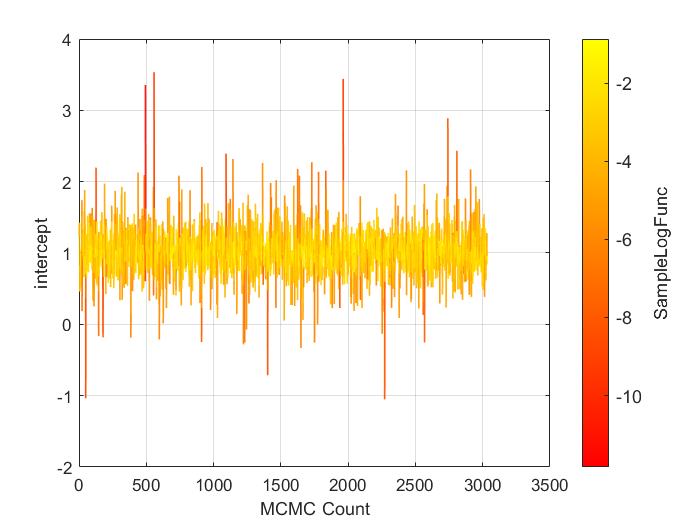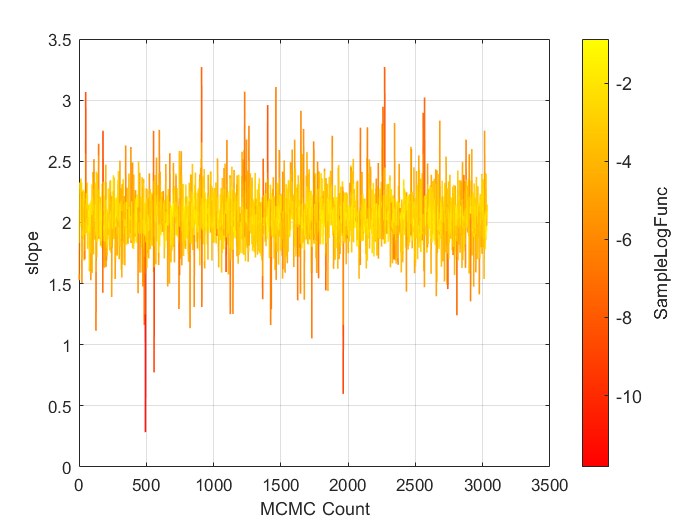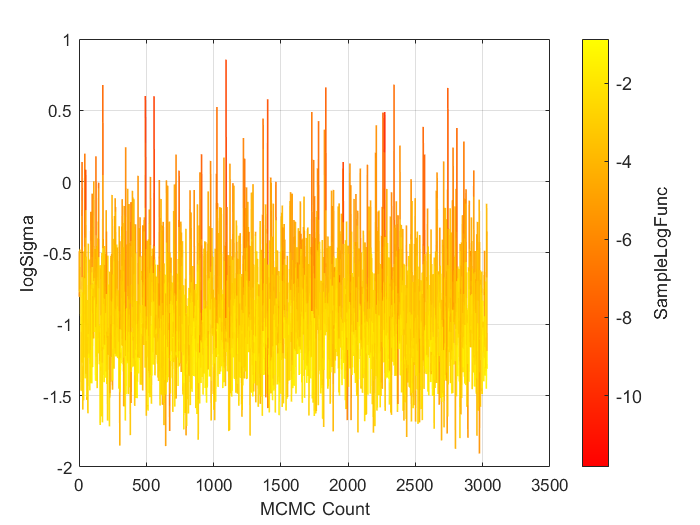% Plot the histogram of the sampled variables.
for colname = sample.df.Properties.VariableNames(2:end)
sample.plot.histogram.make("xcolumns",colname);
xlabel("MCMC Count");
ylabel(colname);
end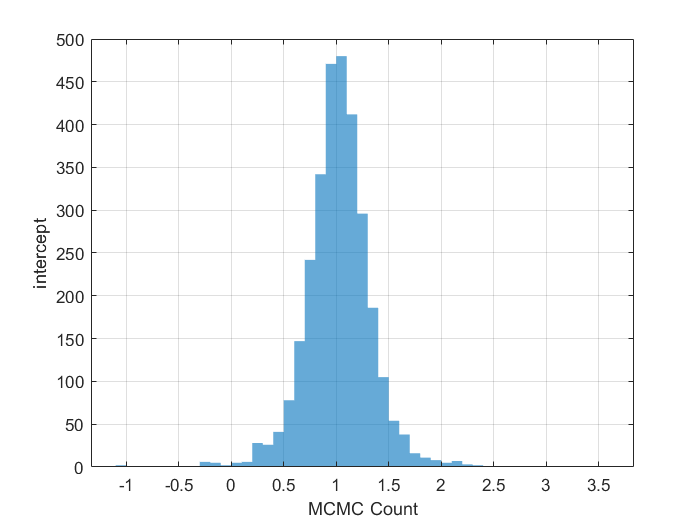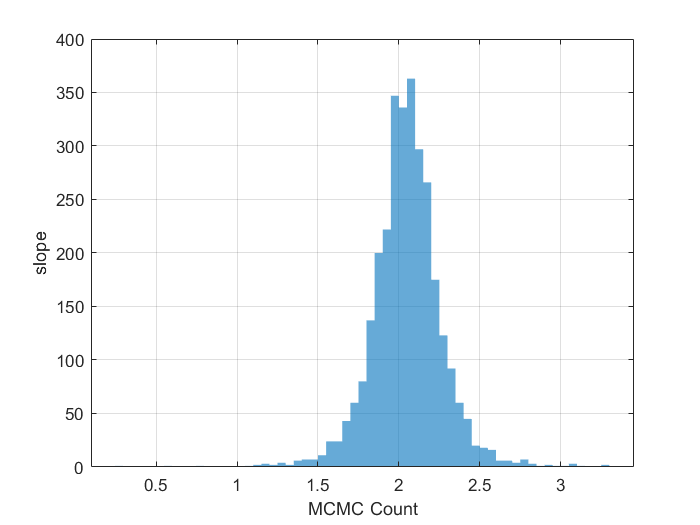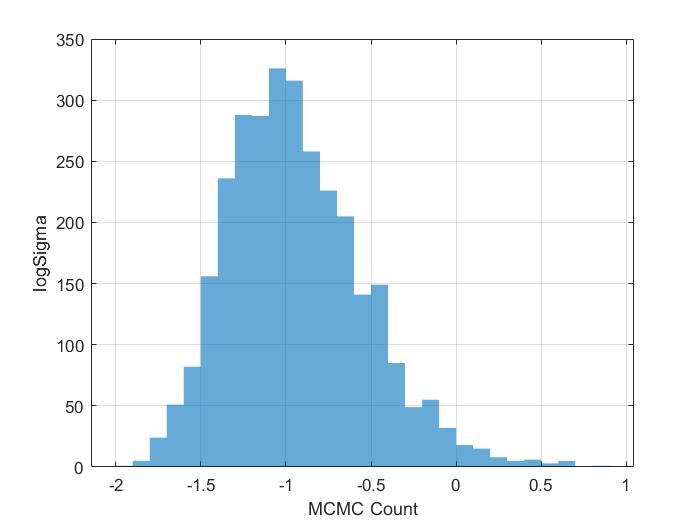## General Guidelines on the ParaMonte visualization tools

===================================================================
In the following sections, we will discuss some of the postprocessing and visualization tools that automatically ship with the ParaMonte library. However, this is just the tip of the iceberg. For more detailed instructions on how to use the individual visualization tools of the ParaMonte library, visist the following MATLAB live scripts,
line / scatter plots
density plots
other plots
Finally, lets take a look at the mean inferred values of the parameters.
sample.df.Properties.VariableNames(2:4)
ans = 1×3 cell
'intercept' 'slope' 'logSigma'
mean(sample.df{:,2:4})
ans = 1×3
1.022976101134784 2.048782241941972 -0.937985291135638
std(sample.df{:,2:4})
ans = 1×3
0.323266011393424 0.219215523337699 0.401223654160306
Our initial guess for the parameter values was not too far from the best-fit values! As for the value of sigma, all we need to do is to exponentiate the mean value of logSgima.
sigma = exp(mean(sample.df.logSigma));
disp("sigma (i.e., normal dispersion of data around the mean expected values) = " + num2str(sigma))
sigma (i.e., normal dispersion of data around the mean expected values) = 0.39142
Now, lets make a grid plot of all of the parameters sampled together,
sample.plot.grid.reset("hard"); % reset the grid plot, just in case...
ParaDRAM - NOTE: creating the grid plot object from scratch...
sample.plot.grid.plotType.upper.enabled = false; % disable upper triangle.
sample.plot.grid.plotType.lower.value = "lineScatter"; % let lower triangle be lineScatter plot.
sample.plot.grid.columns = [2,3,4]; % corresponding to "intercept, slope, logSigma
sample.plot.grid.make(); % make grid plot of all parameters.
generating subplot #1: (1,1) out of 9
skipping subplot #2: (1,2) out of 9 skipping subplot #3: (1,3) out of 9 generating subplot #4: (2,1) out of 9
generating subplot #5: (2,2) out of 9
skipping subplot #6: (2,3) out of 9 generating subplot #7: (3,1) out of 9
generating subplot #8: (3,2) out of 9
generating subplot #9: (3,3) out of 9